# AP Physics 2 Practice Test 17

### Test Information10 questions18 minutes

1.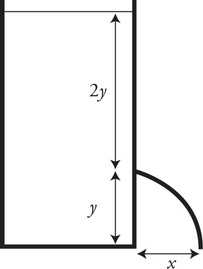A large container of water sits on the floor. A hole in the side a distance y up from the floor and 2y below the surface of the water allows water to exit and land on the floor a distance x away as shown in the figure. If the hole in the side was moved upward to a distance 2y from the floor and y below the surface of the water, where would the water land?

2. A cylinder with a movable piston contains a gas at pressure P = 1 × 105 Pa, volume V = 20 cm3, and temperature T = 273 K. The piston is moved downward in a slow steady fashion allowing heat to escape the gas and the temperature to remain constant. If the final volume of the gas is 5 cm3, what will be the resulting pressure?

3. An equal number of hydrogen and carbon dioxide molecules are placed in a sealed container. The gases are initially at a temperature of 300 K when the container is placed in an oven and brought to a new equilibrium temperature of 600 K. Which of the following best describes what is happening to the molecular speeds and kinetic energies of the gases’ molecules as they move from 300 K to 600 K?

4. A convex lens of focal length f = 0.2 m is used to examine a small coin lying on a table. During the examination, the lens is held a distance 0.3 m above the coin and is moved slowly to a distance of 0.1 m above the coin. During this process, what happens to the image of the coin?

5.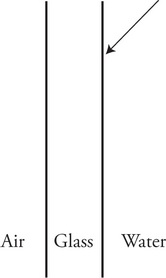Light from inside an aquarium filled with water strikes the glass wall as shown in the figure. Knowing that nwater = 1.33 and nglass = 1.62, which of the following represents a possible path that the light could take?

6.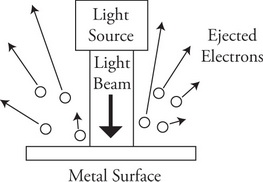A beam of ultraviolet light shines on a metal plate, causing electrons to be ejected from the plate as shown in the figure. The velocity of the ejected electrons varies from nearly zero to a maximum of 1.6 × 106 m/s. If the brightness of the beam is increased to twice the original amount, what will be the effect on the number of electrons leaving the metal plate and the maximum velocity of the electrons?

Number of Electrons Ejected     Maximum Velocity of Ejected Electrons

7.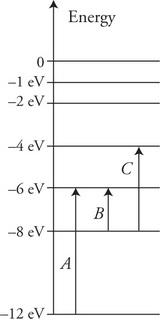Scientists shine a broad spectrum of electromagnetic radiation through a container filled with gas toward a detector. The detector indicates that three specific wavelengths of the radiation were absorbed by the gas. The figure shows the energy level diagram of the electrons that absorbed the radiation. Which of the following correctly ranks the wavelengths of the absorbed electromagnetic radiation?

8.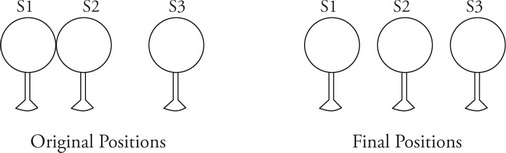Three identical uncharged metal spheres are supported by insulating stands. They are placed as shown in the left figure with S1 and S2 touching. A sequence of events is then performed.

•  S3 is given a negative charge.

•  S1 is moved to the left away from S2.

•  S3 is brought into contact with S2 and then placed back in its original position.

This leaves the spheres in the positions shown in the right figure. Which of the following most closely shows the signs of the final net charge on the spheres?

9.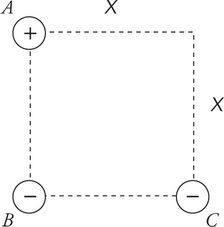Three identical objects with an equal magnitude of charge are placed on the corners of a square with sides of length x as shown in the figure. Which of the following correctly expresses the magnitude of the net force F acting on each charge due to the other two charges?

10.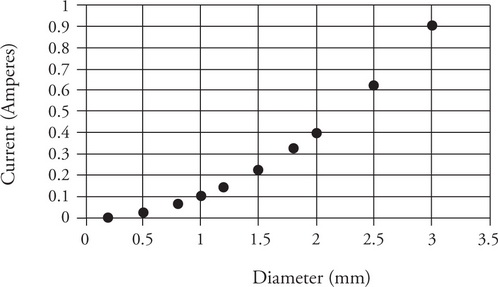In an experiment, a long wire is connected to a battery and the current passing through the wire is measured. The wire is then removed and replaced with new wire of the same length and material but having a different diameter. The figure shows the experimental data graphed with the current as a function of the wire diameter. Which of the following statements does the data support?